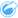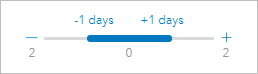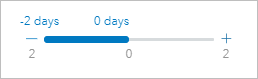# Moving Average

Moving Average calculates average values for a specified window and plots the values on a time series graph. A moving average creates a smoothing effect and reduces noise from daily fluctuations. Moving Average can also be used to impute missing data with estimated values.

## Examples

A stock market analyst is analyzing the value of different stocks. The analyst calculates moving average to track the trends in the stock prices and determine which stocks are growing in value and which stocks are losing value.

An epidemiologist is studying an outbreak of an infectious disease, but her dataset is missing values for several dates. A central moving average is used to calculate estimated values for the missing dates.

## Use the Moving Average capability

Moving Average can only be run using a time series graph with a numeric field on the y-axis.

Complete the following steps to run the Moving Average analysis capability:

1. Create a time series chart using one of the following data combinations:
• One date/time field and one or more number or rate/ratio fields
• One or more date/time fields and one number or rate/ratio field
2. If necessary, click the time series card to activate it. A card is active when the toolbar and Action buttonappear.
3. Click the Action button and choose Moving Average.
4. For Choose number field or Choose date/time field, select the number or date/time field with which you want to calculate moving average. This parameter is only available if the time series graph was created with multiple number fields or multiple date/time fields.
5. For Moving average period, enter the number of days to use in the moving average calculation and adjust the slider if necessary. See Usage notes for more information.
6. Expand Additional options and change the Daily aggregation parameter if necessary.
7. Click Run.

## Usage notes

The Choose a number field parameter is available on time series charts created using two or more numbers on the y-axis. The Choose date/time field parameter is available when two or more date/time fields are used on the time series. These parameters determine which field to use when calculating moving average.

The Moving average period parameter determines how many days are included in the moving average calculation. For example, if you want to calculate a one-week moving average, use a period of seven days. The period must be an odd number. The default period is three days.

The slider in the Moving average period parameter determines which days are used in the calculation. The most common uses of moving average place the calculated value (labeled as day 0 on the slider) as either the endpoint (trailing moving average) or the midpoint (central moving average). By default, Moving Average calculates a central moving average, meaning there is an equal number of data points before and after the value being calculated.You can move the slider to change which days are used in the calculation. Moving the slider to the negative end changes the calculation to a trailing moving average. For example, using the default period of three creates a trailing moving average where the date being calculated and the preceding two days are used in the calculation.You can also use the slider to create a moving average calculation with a custom number of days before and after the day being calculated, rather than using a central or trailing moving average.

Moving average is calculated using a single data point for each day. The Daily aggregation parameter determines how multiple values from a single day are aggregated into a single data point. The aggregation options include average, minimum, and maximum. The default aggregation is average.

The moving average line is added to the time series graph. A new field named n-day moving average (where n is the value of the Moving average period parameter) is added to the input dataset. You can run Moving Average multiple times on the same dataset. Each result will be added to the time series graph and appended to the input dataset.

## Limitations

Moving Average can only be run from a time series graph created using at least one number field.

The Moving average period parameter must be an odd number between 3 and 999.

## How Moving Average works

Moving Average uses a day-based simple moving average (SMA) calculation. The Moving average period parameter and the corresponding slider are used to determine which days are used in the calculation.

The moving average calculation applies the moving average period to dates in your dataset, not calendar days. If a date is missing from your dataset, the next closest date in the dataset will be used, rather than using the consecutive calendar date. Therefore, datasets with large ranges of missing dates may result in inaccurate calculations near the beginning and end of the missing dates.

Dates containing null or missing data are included in the period but subtracted from the value of n (see the equation below). If you do not want to include null or missing data, you can apply a filter to the dataset before running Moving Average. For example, you want to calculate moving average based on business days and exclude null values for weekends. You can apply a filter based on the Day of week subfield for your input date/time field to remove values for Saturday and Sunday.

The data for each day is aggregated using the statistic in the Daily aggregation parameter and then applied to the following formula:

MA0 = (d1+...+dn-1+dn)/(n-x)

where

• MA0 = The moving average for the current date. This date can correspond to any position in the equation (d1 to dn).
• d = The aggregated daily value.
• n = The number of daily values in the moving average calculation (the moving average period).
• x = The number of aggregated daily values within the moving average range that are null. This value can range from 0 to n-1 in a valid calculation. If x = n, moving average will not be calculated for that day.